# Ex 13.3 NCERT Solutions- Surface Areas and Volumes Class 9 Notes | EduRev

## Class 9 : Ex 13.3 NCERT Solutions- Surface Areas and Volumes Class 9 Notes | EduRev

The document Ex 13.3 NCERT Solutions- Surface Areas and Volumes Class 9 Notes | EduRev is a part of the Class 9 Course Mathematics (Maths) Class 9.
All you need of Class 9 at this link: Class 9

Question 1. Diameter of the base of a cone is 10.5 cm and its slant height is 10 cm. Find its curved surface area.
Solution:
Here, diameter of the base = 10.5 cm
Slant height (l) = 10 cm
∴ Curvered surface area of the cone = πrl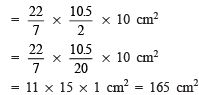Question 2. Find the total surfce area of a cone, if its slant height is 21 m and diameter of its base is 24 m.
Solution:
Here, diameter = 24 m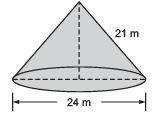⇒ Radius (r) = (24/2)m = 12 m
Slant height (l) = 21 m
∴ Total surface area = πr(r + l)Question 3. Curved surface area of a cone is 308 cm2 and its slant height is 14 cm. Find:  (i) radius of the base and (ii) total surface area of the cone.
Solution:
Here, curved surface area = 308 cm
Slant height (l) = 14 cm

(i) Let the radius of the base be ‘r’ cm.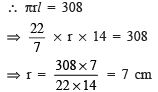Thus, the required radius of the cone is 7 cm.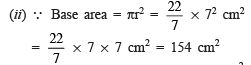and curved surface area = 308 cm2                [Given]
∴ Total surface area = [Curved surface area] + [Base area]
= 308 cm2 + 154 cm2 = 462 cm2

Question 4. A conical tent is 10 m high and the radius of its base is 24 m. Find: (i) slant height of the tent. (ii) cost of the canvas required to make the tent, if the cost of 1 mcanvas is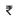70.
Solution:
Here, height of the tent (h) = 10 m
Radius of the base (r) = 24 m (i)
∵ The slant height,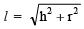∴ The slant height of the tent =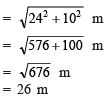Thus, the required slant height of the tent is 26 m.

(ii) ∵ Curved surface area of the cone = πrl
∴ Area of the canvas required =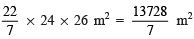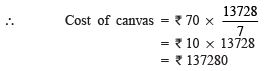Question 5. What length of tarpaulin 3 m wide will be required to make conical tent of height 8 m and base radius 6 m? Assume that the extra length of material that will be required for stitching margins and wastage in cutting is apπroximately 20 cm (Use π = 3.14).
Solution:
Here, Base radius (r) = 6 m
Height (h) = 8 m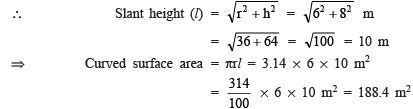∴ Area of the canvas (tarpaulin) required to make the tent = 188.4 m
Let the length of the tarpaulin = ‘L’ m
∴ Length x Breadth = 188.4
⇒ L x 3 = 188.4
⇒ L= (188.4/3) = 62.8 m
Extra length of tarpaulin  for margins = 20 cm = (20/100) m = 0.2 m
Thus, total length of tarpaulin required = 62.8 m + 0.2 m = 63.0 m

Question 6. The slant height and base diameter of a conical tomb are 25 m and 14 m respectively.
Find the cost of white washing its curved surface at the rate of210 per 100 m2.
Solution:
Here, Base radius (r) = (14/7) = 7 m
Slant height (l) = 25 m
∴ Curved surface area = πrl = (22/7) x 7 x 25 m
= 22 x 25 m2 = 550 m
Cost of white washing:
Rate of whitewashing =210 per 100 m2
∴ Cost of whitewashing for 550 m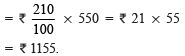Question 7. A joker’s cap is in the form of a right circular cone of base radius 7 cm and height 24 cm. Find the area of the sheet required to make 10 such caps.
Solution
: Here, Radius of the base (r) = 7 cm  height (h) = 24 cm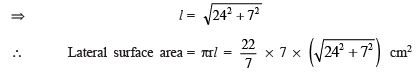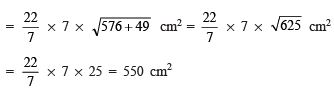⇒ Lateral surface area of 10 caps = 10 x 550 cm= 5500 cm
Thus, the required area of the sheet = 5500 cm

Question 8. A bus stop is barricaded from the remaining part of the road, by using 50 hollow cones made of recycled cardboard. Each cone has a base diameter of 40 cm and height 1 m. If the outer side of each of the cones is to be painted and the cost of painting is 12 per m2, what will be the cost of painting all these cones? (Use π = 3.14 and take √1.04= 1.02)
Solution:
Here, ∵ Diameter of the base = 40 cm

∴ Radius (r) = 40/2 cm = 20 cm = 10/100 m

= 2/10 m = 0.2 m

Height (h) = 1 m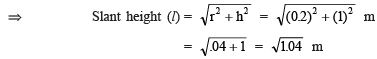= 1.02 m [∵ √1.04= 1.02             (Given)]
Now, curved surface area = πrl

⇒ Curved surface area of 1 cone = 3.14 x 0.2 x 1.02 m2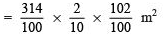⇒ Curved surface are of 50 cones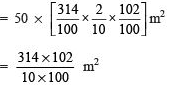Cost of painting
∵ Rate of painting = ₹ 12 per m2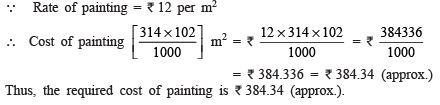SURFACE AREA OF A SPHERE

A solid generated by revolving a circular lamina about any of its diameters, is called a sphere. If ‘r’ be its radius, then its surface area = 4πr2.

For the following πroblems, assume π = (22/7), unless stated otherwise.

Offer running on EduRev: Apply code STAYHOME200 to get INR 200 off on our premium plan EduRev Infinity!

## Mathematics (Maths) Class 9

190 videos|233 docs|82 tests

,

,

,

,

,

,

,

,

,

,

,

,

,

,

,

,

,

,

,

,

,

;